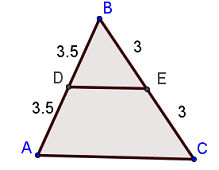# Mid-Segment of a Triangle Worksheets

What is a Mid-Segment of a Triangle? The line segment formed by joining the midpoint of any two sides of the triangle is known as the mid-segment of a triangle. To put it simply, the midsegment essentially divides the two sides of a triangle equally. The midpoint of this midsegment divides both sides into equal halves.In the figure shown above, we can see that the line segment DE is the midsegment of the triangle ABC. Point is diving side BC into equal halves, and the point D is dividing side AB into equal halves. However, the one side that the midsegment doesn’t interact with is the base of the triangle. The midsegment of a triangle has several properties; some of them are mentioned below: The midsegment is parallel to the base of the triangle. The midsegment of the triangle is exactly half the length of the base. The perimeter of the triangle formed by the midsegment and the two half sides are equal to the one-half the original perimeter of the triangle. The two half-sides and the triangle formed by the midsegment are exactly one-fourth of the area of the original triangle. The angles of the two half sides and the triangle formed by the midsegment are of the same measurement as the original triangle.

• ### Basic Lesson

Guides students through solving Mid-Segment of a Triangle. As the mid-segment of a triangle joins the midpoints of two sides of a triangle such that its length is half the length of the third side of the triangle.

• ### Intermediate Lesson

Demonstrates the concept of advanced skill while solving Mid-Segment of a Triangle. Given AC = 5, CB = 3, AB = 4. A, B & C are midpoints. Find the perimeter of △ PQR.

• ### Independent Practice 1

A really great activity for allowing students to understand the concepts of the Mid-Segment of a Triangle.

• ### Independent Practice 2

Students use Mid-Segment of a Triangle in 20 assorted problems. The answers can be found below.

• ### Homework Worksheet

Students are provided with 12 problems to achieve the concepts of Mid-Segment of a Triangle.

• ### Skill Quiz

This tests the students ability to understand Mid-Segment of a Triangle.